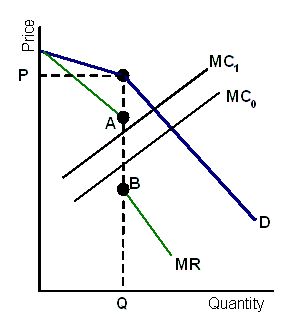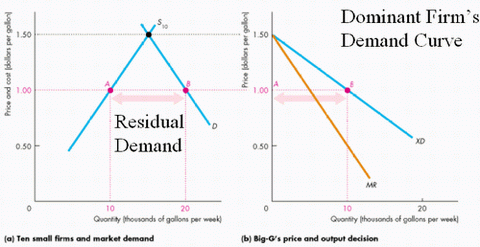### Why should I choose AnalystNotes?

AnalystNotes specializes in helping candidates pass. Period.

##### Subject 8. Oligopoly

Literally, oligopoly means "few sellers." This market structure is characterized by:

• A small number of rival firms. The firms are interdependent because each is large relative to the size of the market. The decisions of a firm often influence the demand, price, and profit of rivals, and an oligopolist must consider the potential reaction of rivals.

• High entry barriers into the market. Either natural or legal barriers to entry can create oligopoly.

• Economies of scale are probably the most significant entry barrier here. Achieving minimum per-unit cost is required, and thus a small number of large-scale firms will be able to produce the entire market demand for the product. This is what distinguishes an oligopoly from a monopolistic competitive market.
• A legal oligopoly might arise even where demand and costs leave room for a larger number of firms.

In short, an oligopoly is competition among the few.

Pricing Strategies

• Like a monopolist, an oligopolist faces a downward-sloping demand curve and seeks to maximize profit, not price.
• Unlike a monopolist, an oligopolist cannot determine the product price that will deliver maximum profit simply by estimating market demand and cost conditions.

A key factor here is the pricing behavior of close rivals, or interdependence between firms. This means that each firm must take into account the likely reactions of other firms in the market when making pricing decisions. Because the reactions of those rivals cannot be determined, the precise price and output that will emerge under an oligopoly cannot be determined. Only a potential range of prices can be indicated.

There are three basic pricing strategies.

1. The assumption of pricing interdependence is that firms will match a price reduction and ignore a price increase. The idea is that if a firm raises prices, other firms won't follow, because they won't worry about losing market share to a firm that is raising its prices. However, if the firm lowers its prices, other firms will respond by lowering their prices also, since they don't want to lose market share.

The demand curve that a firm believes it faces has a kink at the current price P and quantity Q.The kinked demand curve can be thought of as two demand curves.

• Above the price P, an individual firm is afraid of putting up prices. A price increase would, it assumes, not be matched by competitors, hence the demand curve above P is elastic. It will be remembered that if demand is elastic and price rises, revenue falls.
• Similarly, a price fall has the same effect on revenue. This time the firm imagines that dropping its own price leads to others dropping theirs. Overall, quantity demand increases as the demand curve slopes down, but the increase is less than proportionate. That is the demand curve below price P is inelastic.

The kink in the demand curve means that the MR curve is discontinuous at the current quantity - shown by the gap AB in the figure.

Fluctuations in MC that remain within the discontinuous portion of the MR curve leave the profit-maximizing quantity and price unchanged.

For example, if costs increased so that the MC curve shifted upward from MC0 to MC1, the profit-maximizing price and quantity would not change.

The beliefs that generate the kinked demand curve are not always correct and firms can figure out this fact. If MC increases enough, all firms raise their prices and the kink vanishes.

2. The assumption of the Cournot model is that a firm will embrace another's output decisions in selecting its profit-maximizing output but that decision is fixed. This means that each firm is naively conjecturing that should either one of them alter their output decisions, the other will not react.

Example

Assume there are 2 firms. The market demand takes the following form: P = 30 - Q, where Q = Q1 + Q2 and Q1 = Q2 (i.e., industry output constitutes firm 1 and 2's output respectively and both firms share the market).
Also assume that average cost (AC) and marginal cost (MC): AC = MC = 12.

Firm 1's total revenue (TR) is P x Q1 = (30 - Q) x Q1 = [30 - (Q1 + Q2)] x Q1 = 30 x Q1 - Q12 - Q1 x Q2.
Firm 1's MR is thus MR1 = 30 - 2Q1 - Q2
If MC = 12, then Q1 = 9 - 1/2 x Q2. This is Firm 1's reaction curve.
For Firm 2, Q2 = 9 - 1/2 x Q1.
Solving for Q1, we find Q1 = 6, Q2 = 6 and P = 18.

This equilibrium can be compared with that of perfect competition and monopoly.

• Perfect Competition: Firms set prices equal to MC. So P = 12 and Q = 18.
• Monopoly: TR = PQ = (30 - Q)Q = 30Q - Q2, MR = 30 - 2Q. As MC = 12 = MR, Q = 9 and P = 21.

We can see that 2 firms operating under Cournot assumptions offer a better welfare outcome than under monopoly.If the number of firms increases, then the Cournot equilibrium approaches the competitive equilibrium.

3. Nash equilibrium is a concept of game theory where a firm does what is best for itself after it takes into account other firms' actions. For example, McDonald's charges \$2.99 for a Value Meal based on what Burger King and Wendy's are charging for a similar menu item. McDonald's would reconsider its pricing if its rivals were to change their prices.

An Oligopoly Price-Fixing Game

Collusion is the opposite of competition. It involves cooperative actions by sellers to turn the terms of trade in favor of the group and against buyers.

If there is no collusion and each oligopolist act independently, seeking to maximize profits by offering consumers a better deal than its rivals, the market price would be driven down to its lowest level and firms would be just able to cover their per-unit cost. This is like a pure competitive market.

However, there is a strong incentive for oligopolists to collude, agreeing to raise price and to restrict output. They can form a cartel (such as OPEC) or they can collude without such a formal organization. In this case, the highest price occurs.

Oligopolists have a strong incentive to collude since they can profit by restricting output and raising price. There are six major factors that affect the chances of successful collusion:

• The number and size distribution of sellers
• The similarity of the products
• Cost structure
• Order size and frequency
• The strength and severity of retaliation
• The degree of external competition

Optimal Price and Output

There is no single optimum price and output analysis that fits all oligopoly market situations. Consider a dominant firm model where the market consists of a dominant firm and some fringe firms. The dominant firm becomes the price maker. It operates as a monopoly, faces a residual demand curve, and chooses price and output to maximize its profit (MR = MC). Other firms are price takers or followers.

Example

The following figure shows a dominant firm industry. On the left are 10 small firms and on the right is one large firm.• The demand curve, D, is the market demand curve and the supply curve, S10, is the supply curve of the 10 small firms.
• At a price of \$1.50, the 10 small firms produce the quantity demanded. At this price, the large firm would sell nothing.
• But if the price was \$1.00, the 10 small firms would supply only half the market, leaving the rest to the large firm.
• The demand curve for the large firm's output is the curve XD on the right. The large firm can set the price and receives marginal revenue that is less than that price along the curve MR.
• The large firm maximizes profit by setting MR = MC. Let's suppose that the marginal cost curve is MC in the figure. The profit-maximizing quantity for the large firm is 10 units. The price charged is \$1.00.
• The small firms take this price and supply the rest of the quantity demanded.

User Comment
Yass0707 i don't understand how can get MR for the 1st firm from TR.

If TR=30Q1 - Q1(square2) - Q1Q2
how we can get MR=30 - 2Q1 - Q2
Yass0707 never mind, i figured out :)
kcanales4 I can't seem to figure out how MR=30-2Q1-Q2. Can someone please explain? Thanks!
Bududeen You differentiate! That is find the first derivative
schweitzdm Could someone please explain how you find Q1 = 6 from that same example? This reading is causing a major headache.
robbiecow Sub Q1 = 9 -.5Q2 into Q2 = 9 - .5*(9 - .5Q2) ... Q2 = 6, which also means that Q1 = 6.
abellochs are they serious with all those derivatives and differentiation?? Just spent an hour remembering all that. Can't wait to use it in the real world. SMH
walterli there is NO COLLUSION!
dsndea10 under cournot model this step is wrongly calculated in the above notes
Firm 1's total revenue (TR) is P x Q1=[30 - (Q1 + Q2)] x Q1
= 30 x Q1 - Q12 - Q1 x Q2.